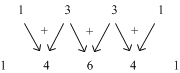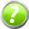x2
Introduction
( )
Types of Arrangements
=
Two More Methods
=
Some Applications

# Section 2.3 – Pascal's Triangle

Quick Navigation Video Lectures Exercises

In the Analysis part of the last section's exercises, you saw an interesting pattern in the coefficients: The numbers that you get from the Binomial Theorem for a polynomial are mirror images of each other, i.e. the first and last are the same, the second and the second to the last are the same, etc. There's an even bigger pattern that you get from looking at the values as the polynomial's degree increases. The table below has the expansions of the first several binomials.

BinomialPolynomial Expansion
(x + y)01
(x + y)1x + y
(x + y)2x2 + 2xy + y2
(x + y)3x3 + 3x2y + 3xy2 + y3
(x + y)4x4 + 4x3y + 6x2y2 + 4x3y + y4

Now, if I look at the Polynomial Expansion row, but just write down the coefficients, I'll get this:

1

11

121

1331

14641

That may not look very interesting at first but, for example, take a look at the fourth and fifth lines. Notice how if you add together the 1 and the 3 at the beginning of the fourth line you get 4 (the second number on the fifth line). And if you add together the 3 and the 3 on the fourth line you get 6 (the third number on the fifth line). The whole pattern is laid out in the diagram below. Each row starts and ends with a 1 and the other numbers are the sum of two consecutive numbers from the previous line.Using this argument, the next row has to be

 1 (1 + 4) (4 + 6) (6 + 4) (4 + 1) 1 1 5 10 10 5 1

If you compare those numbers to the values that we get from the Binomial Theorem, you'll see that they correspond to the coefficients of (x + y)5.

 1 5 10 10 5 1 5C5 5C4 5C3 5C2 5C1 5C0

1

11

121

1331

14641

15101051

. . .

The triangular array of numbers where each row is made from adding the consecutive numbers on the previous row is called Pascal's Triangle after the 17th century French mathematician, Blaise Pascal. Because the values in the array correspond to the coefficients of binomial expansion, this gives us an even quicker method than the Binomial Theorem for expanding a polynomial. If you need the coefficients of (x + y)7, for example, all you have to do is write out the first eight rows of the triangle and you'll have the numbers that you need. (You need eight rows to get the coefficients for (x + y)7 because the rows of the triangle start with (x + y)0.) A quick way to be sure you're using the correct row is to look at its second number. That value always corresponds to the degree of the polynomial. For example, the last line in the example above has 5 as its second number so it corresponds to the coefficients of (x + y)5.

# Exercises

### Exercises= show solution= get hint

1. Write out the first ten rows of Pascal's Triangle.
2. What polynomial's coefficients does the tenth row in the triangle correspond with?
3. Using Pascal's Triangle, write out the expansion of $(x+y)^8$.
4. Using Pascal's Triangle, write out the expansion of $(x-y)^7$.